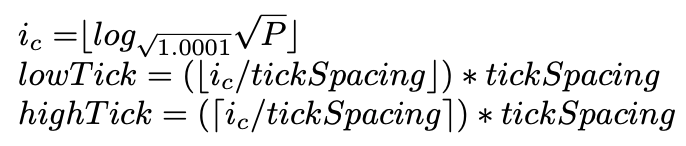# How to convert a price to a tick that can be initialized

#### Converting a price to a tick that can be initialized1 is done using the following formula:#### To convert a price to a tick that can be initialized:

1. Turn the price into a sqrtPrice.

To turn the price into a sqrtPrice, you will have to get the ratio value with an example of the pool ratio.3 This is done using 1 token of ETH (token1) and the price of the USDC (token0) desired for the chosen range.

2. Select a price

``TickLow_USDC = 1000``
1000 USDC for 1 ETH

3. Converting the price into the lowest decimal format:

``TickLowFormatted =  TickLow_USDC*10decimal token0``
USDC is token0 which has 6 decimals.

4. Encode the sqrtPrice Ration

Take 1 ETH in wei (lowest decimal ie. 1018), and the USDC price formatted. Using them as the pool ratio reserves3

``Token1ReserveRatio= 1000000000000000000``
``Token0ReserveRatio= 1000000000``

Next, take the token 1 amount and divide by the token 0 amount, to get the sqrtPriceRatio.

`sqrtPriceRatio = (Token1ReserveRatio/Token0ReserveRatio)`

5. Get the tick at the sqrtPrice:

`tickForPrice = floor(log(sqrtPriceRatio, 1.0001))  `

6. This will give you the tick for the price `207243` after taking the rounded-down floor value and removing decimals.

7. Adjust the tick to the tick spacing of the pool to get a valid initializable tick.

Take the tick for the chosen price divided by the tick spacing of the pool and the rounded-down floor value5 of the pool.

Next, multiply the floored value by the tick spacing.

``closestLowTick = floor( tick / tickSpacing ) * tickSpacing``

Note: For the closest high tick, you will take the ceiling of the `tick / tickSpacing`. This will give the closest tick range that includes the chosen price.

Footnotes:

1. A tick that can be initialized must be evenly divisible by the tick spacing of the pool. Initialized ticks are used for liquidity positions. Learn more about this here: Uniswap v3 whitepaper section 6.1 paragraph 8, and image 6.8

2. SqrtPrice is the current price of the pool. Check out the Math Primer for more.

3. SqrtPriceRatio / pool ratio reserves. This is the ratio of tokens that would be in the pool. Imagine these two numbers as the initial liquidity deposit that sets the price of the pool. Check out the Math Primer Part 2 for more.

Updated